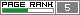Sunday, June 05, 2005

The Easom Function

Hello!!

Here I'm again to show another result that I got with my ES.

The function that I used this time was the Easom Function:

F(x1,x2) = -cos(x1)*cos*x2)*exp(-(x1-pi)^2 - (x2-pi)^2)
x1 = [-100:100]
x2 = [-100:100]

ES Configuration:

Population = 60
Offspring = 60 (I used the (mu+mu)ES )
Generation = 5000
Simulation Time = 6.99 s
The problem is to minimize the function above. The global minimum is -1 with x1 = pi and x2 = pi.

Below I show you my results.

Best initial values:
F(x1,x2) = -2.0079019279809812e-027
x1 = 4.405669730345565
x2 = 10.71696945361343

Best final values:
F(x1,x2) = -0.99999978685693391
x1 = 3.141922312441527
x2 = 3.1414098408067366

Here we have the Easom Function's graph:A side view:Here you see the evolution of the ES. The horizontal axis is the number of generations and the vertical axis is the best fitness value.Well, I think that my ES found very good solutions to the Easom Function. :D

See You!!

Nosophorus